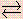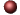Types of Chemical ReactionsExamples ofMultiple Choice Questions

1.
What alkaline earth metal is located in period 3?
(a) Li
(b) Na
(c) Ca
(d) Mg
(e) Sr

2.
Which of the following is classified as a metal?
(a) Ge
(b) As
(c) F
(d) V
(e) Ar

3.
Which of the following is a weak acid?
(a) H2SO4
(b) HClO3
(c) HF
(d) HCl
(e) HNO3

4.
Which one of the following is likely to be the most soluble base?
(a) Ca(OH)2
(b) Cu(OH)2
(c) Ga(OH)3
(d) Zn(OH)2
(e) Zr(OH)3

5.
Which one of the following statements is TRUE?
(a) One mole of any acid will ionize completely in aqueous solution to produce one mole of H+ ions.
(b) Solutions of weak acids always have lower concentrations of H+ than solutions of strong acids.
(c) There are several common acids that are insoluble.
(d) All of the IA and IIA metal hydroxides are soluble.
(e) All weak acids are insoluble.

6.
Consider the following reaction:
NH3(g) + H2O(l)NH4+(aq) + OH-(aq)
Which one of the following statements is false?
(a) The double arrows indicate that ammonia, NH3, is only very slightly soluble in water.
(b) The reaction is reversible.
(c) When ammonia is added to water, NH4+ and OH- ions are produced in a 1:1 ratio.
(d) When solutions of NH4Cl and NaOH are mixed, some ammonia is produced.
(e) Ammonia is considered to be a weak base.

7.
Which one of the following salts is insoluble?
(a) NH4Cl
(b) Ca(NO3)2
(c) BaCO3
(d) Na2S
(e) Zn(CH3COO)2

8.
What salt is formed in the following acid/base reaction?
HClO3 + Ba(OH)2(a) BaCl2
(b) ClOBa
(c) H2O
(d) BaClO3
(e) Ba(ClO3)2

9.
The precipitate formed when barium chloride is treated with sulfuric acid is _______ .
(a) BaS2O4
(b) BaSO3
(c) BaSO2
(d) BaSO4
(e) BaS

10.
The spectator ion(s) in the following reaction is/are:
Na2CO3(aq) + Ba(NO3)2(aq)BaCO3(s) + 2NaNO3(aq)
(a) Na+ and Ba2+
(b) Ba2+ and CO32-
(c) CO32- and NO3-
(d) Na+ only
(e) Na+ and NO3-

11.
Which one of the following statements is FALSE?
(a) For the reaction of a strong acid with a strong soluble base, the net ionic equation is always
H+ + OH-H2O
(b) "Spectator ions" appear in the total ionic equation for a reaction, but not in the net ionic equation.
(c) HF, HCl, and HNO3 are all examples of strong acids.
(d) Titration is a process which can be used to determine the concentration of a solution.
(e) In a neutralization reaction, an acid reacts with base to produce a salt and H2O.

12.
What is the net ionic equation for the acid-base reaction that occurs when acetic acid and potassium hydroxide solutions are mixed?
(a) H+(aq) + OH-(aq)H2O(l)
(b) H+(aq) + KOH(s)K+(aq) + H2O(l)
(c) CH3COOH(aq) + KOH(s)KCH3COO(aq) + H2O(l)
(d) CH3COO-(aq) + H+(aq) + K+(aq) + OH-(aq)K+(aq) + CH3COO-(aq) + H2O(l)
(e) CH3COOH(aq) + OH-(aq)CH3COO-(aq) + H2O(l)

13.
What is the net ionic equation for the acid-base reaction that occurs when nitric acid is added to copper(II) hydroxide?
(a) H+(aq) + OH-(aq)H2O(l)
(b) 2H+(aq) + Cu(OH)2(s)Cu2+(aq) + 2H2O(l)
(c) 2HNO3(aq) +Cu(OH)2(s)Cu(NO3)2(s) + 2H2O(l)
(d) 2H+(aq) + 2NO3-(aq) + Cu2+(aq) + 2OH-(aq)Cu(NO3)2(s) + 2H2O(l)
(e) 2H+(aq) + 2NO3-(aq) + Cu2+(aq) + 2OH-(aq)Cu2+(aq) + 2NO3-(aq) + 2H2O(l)

14.
Which of the following statements is FALSE given the following net ionic equation?
H3PO4(aq) + 3OH-(aq)PO43-(aq) + 3H2O(l)
(a) If all the water evaporated away, the salt remaining could possibly be Na3PO4.
(b) The acid, H3PO4, is a weak electrolyte.
(c) The base involved must be a strong soluble base.
(d) This is classified as a neutralization reaction.
(e)This could be the net ionic equation for H3PO4 reacting with Al(OH)3.

15.
Which of the following statements is FALSE given the following net ionic equation?
2H+(aq) + Cu(OH)2(s)Cu2+(aq) + 2H2O(l)
(a) If all the water evaporated away, the salt remaining could possibly be CuS.
(b) The acid involved must be a strong electrolyte.
(c) The base, Cu(OH)2, is an insoluble base.
(d) This could be the net ionic equation for HNO3 reacting with Cu(OH)2.
(e) This is classified as a neutralization reaction.

16.
Determine the oxidation number of carbon in K2CO3.
(a) 0
(b) +2
(c) +4
(d) -2
(e) some other value

17.
Which assignment of oxidation number is INCORRECT for the blinking element?
(a) K2Cr2O7; +6
(b) NH3; +3
(c) H2PO2-; +1
(d) SeO32-; +4
(e) Cu(NO3)2; +2

18.
Consider the following reaction:
4NH3 + 5O24NO + 6H2O
The element being oxidized and the oxidizing agent are:
(a) N and NH3
(b) N and O2
(c) O and NH3
(d) O and O2
(e) H and NH3

19.
Given that the Activity Series is: Na>Mg>Cu>Ag>Au, which one of the following answers represents the ions that would not be displaced from aqueous solution (reduced) by metallic magnesium?
(a) Na+
(b) Cu2+
(c) Cu2+ and Au+
(d) Cu2+, Ag+ and Au+
(e) Na+, Cu2+, Ag+ and Au+

20.
Which name/formula combination is WRONG?
(a) phosphorous acid / H3PO4
(b) nitrogen oxide / NO
(c) acetate ion / CH3COO-
(d) sodium chromate / Na2CrO4
(e) calcium hypobromite / Ca(BrO)2

21.
Which name/formula combination is WRONG?
(a) chlorous acid / HClO2
(b) dinitrogen tetroxide / N2O4
(c) ammonium nitrate / NH4NO3
(d) copper(II) periodate / CuIO4
(e) potassium permanganate / KMnO4

1. (d) 2. (d) 3. (c) 4. (a) 5. (b) 6. (a) 7. (c) 8. (e) 9. (d) 10. (e) 11. (c) 12. (e) 13. (b) 14. (e) 15. (a) 16. (c) 17. (b) 18. (b) 19. (a) 20. (a) 21. (d)Click here to return to the top.Choose your next chapter:
Fundamentals of Chemistry | Chemical Formulas & Composition Stoichiometry | Chemical Equations & Rxn Stoichiometry | Types of Chemical Reactions |
| Atomic Structure | Chemical Periodicity | Chemical Bonding | Molecular Structure/Covalent Bonding Theories| Molecular Orbital Theory |
| Acids/Bases/Salts - Theory & Rxns | Acids/Bases/Salts - Calculations (including balancing redox rxns) | Gases | Solids & Liquids | Solutions |
| Thermodynamics | Kinetics | Equilibrium | Aqueous Equilibrium - Acids/Bases/Salts | Aqueous Equilibrium - Buffers & Titrations |
| Aqueous Equilibrium - Slightly Soluble Salts | Electrochemistry | Metallurgy | Metal Properties & Rxns | Nonmetals & Metalloids |
| Coordination Compounds | Nuclear Chemistry | Organic Chem - Formulas/Names/Properties | Organic Chem - Shapes/Rxns/Biopolymers |To report any corrections, please e-mail Dr. Wendy Keeney-Kennicutt.